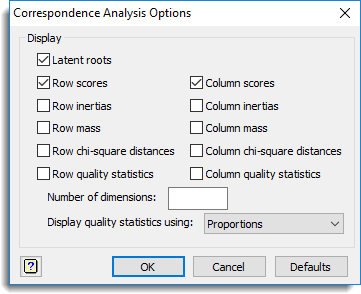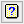1. Home
2. Correspondence Analysis Options

# Correspondence Analysis Options

This selects which results should be printed to the Output window by a correspondence analysis.## Display

Specifies which items of output are to be displayed in the Output window.

 Latent roots The roots (together with the roots expressed as percentages and cumulative percentages) Row scores The scores for the rows of the data matrix Column scores The scores for the columns of the data matrix Row inertias The inertias for the rows of the data matrix Column inertias The inertias for the columns of the data matrix Row mass The row masses Column mass The column masses Row chi-square distances The row chi-square distances Column chi-square distances The column chi-square distances Row quality statistics The quality statistics for the rows Column quality statistics The quality statistics for the columns

## Number of dimensions

Specifies the number of latent roots to display in the output. If this field is left blank then all dimensions will be displayed.

## Display quality statistics using

Controls the representation of the columns of proportions for the quality statistics. These can be displayed as Proportions, Percentages or as Tenths of a percent.

## Action IconsClear Clear all fields and list boxes.Help Open the Help topic for this dialog.